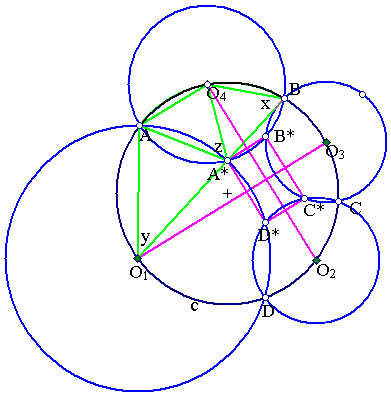##Miquel's theorem on four intersecting circles

Consider four points A, B, C, D on a circle (c) and four other circles passing through two consecutive points out of these four. Then the second points of intersection, A*, B*, C*, D*, of these circles lie on another circle d. Hint. The proof is an easy exercise in measuring the angles of the quadrangle A*B*C*D*.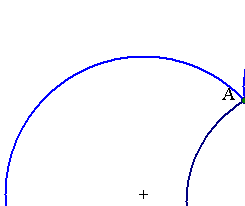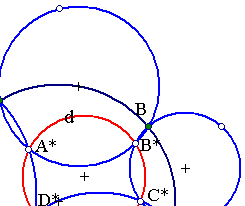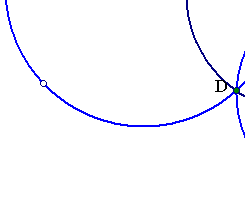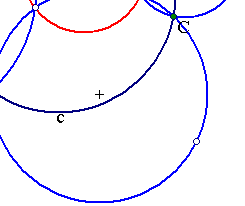In the document Miquel2.html you can find a variant of the theorem, where one of the circles becomes a line. In the document Miquel_Point.html you can find another theorem on two intersecting lines.
The figure below shows a particular case, in which the centers of the four circles lie also on circle (c). Then the other intersection points build a rectangle. A clue fact is that with the angles indicated (z+(pi-y)/2)=(pi-x), which implies that {B,A*,O1} are collinear.